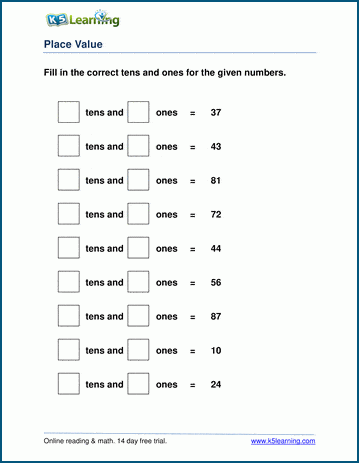# 1st Grade Math Worksheets: Place Value

## Grade 1 place value worksheets

Our grade 1 place value worksheets help students understand our base 10 number system.  Activities include tens and ones, rounding, building 2 digit numbers and changing back and forth between "expanded form" and "normal form".  These worksheets focus on 2 digit numbers (numbers less than 100).

## Tens & Ones Worksheets

Example
Identify tens and ones
__ tens & __ ones = 42
Combine tens and ones
4 tens & 2 ones = __
Identify a digit's place value  42 -> 4 tens

## Building 2-digit numbers

Build a number from tens and ones 70 + 5 = __
Build a number from tens and ones - missing addend 70 + ___ = 75

## Rounding Worksheets

Rounding to the nearest 10 28 -> __

## Expanded Form / Normal Form

Write numbers in expanded form
57 =  5 x 10 + 7
Write numbers in normal form  5 x 10 + 3  = __Sample Grade 1 Place Value Worksheet

## More place value & rounding worksheets

Explore all of our  place value worksheets, from base ten blocks to 6 digit numbers in expanded form, and our  rounding worksheets, ranging from rounding to the nearest 10 to rounding in the millions.

## What is K5?

K5 Learning offers reading and math worksheets, workbooks and an online reading and math program for kids in kindergarten to grade 5.  We help your children build good study habits and excel in school.